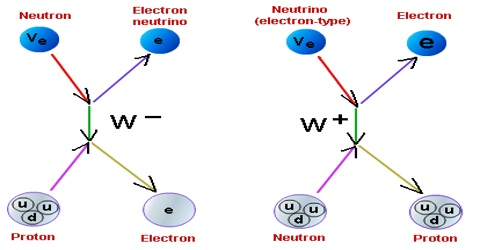Physics

# Weak Nuclear Force: Definition in DynamicsWeak nuclear force: There are some elements found in nature which disintegrate spontaneously (for example; uranium, thorium etc). These nuclei are called radioactive nuclei. Three different types of rays or particles are emitted from radioactive nucleus. These are called alpha rays (α-rays), beta rays (β-rays) and gamma rays (γ-rays).

When beta particles or rays are emitted from radioactive nucleus, then energy is also emitted along with it. But from experimental result it is observed that the amount of energy released from the nucleus is more than the kinetic energy of beta particles. Naturally, question arises; if β-particle carries a little or small portion of energy then where the rest of the energy remains? In 1930 W. Pauli proposed that rest of the energy is carried by another type of particles which air emitted along with β-particles. These particles are called neutrino. The emitted of β-particle, and neutrino particles is due to a fundamental force called weak nuclear force. This force is much weaker than strong nuclear force or electromagnetic force. Disintegration of many nuclei is caused due to this force.

Weak Nuclear Force can transform a neutron into proton or proton into neutron. Weak nuclear forces act between quarks and leptons both. Weak interaction is responsible for the flavor change of quarks and leptons. The significance of weak nuclear force in flavor change of quarks makes it the interaction indulged in many decay phenomenons of nuclear particles which need a change of quark from one type to another.

Weak nuclear force is of two types:

• Charged current Nuclear Force and
• Neutral current Nuclear Force.